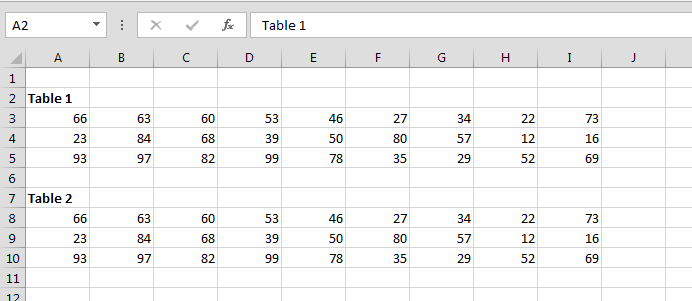# Change all the cells in the ActiveSheet to values using VBA in Microsoft Excel

In this chapter you will learn how to change all the cells in the active sheet to values using VBA in Microsoft Excel.

Let’s take an example and understand how we write the VBA code for change the cells value in Active sheet.

How to change all the cells value in the Active sheet?

We have data in Excel, in which we want the replace all the cells value with only a cell value.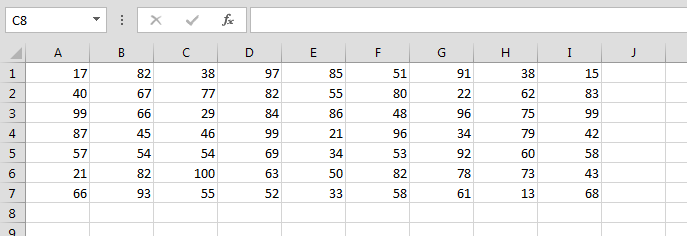• Press Alt+F11 key to open the Visual Basic Application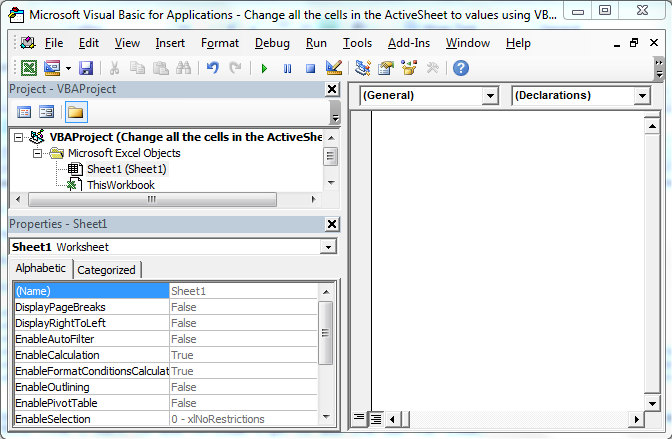• In VBAProject Double click on Sheet 1
• Enter the below given VBA Code

```

Sub Values_1()

With ActiveSheet.UsedRange

Range("A1:I7").Value = Range("A2").Value

End With

End Sub
```

• To run the code press F5 key
• Cell A2 value will get update in the define range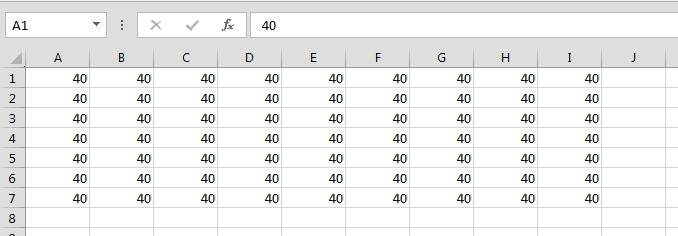How the change the value from first table to second table?

We have 2 tables, 1st table’s range is A3:I5, and 2nd table range is A8:I10. We want to replace the value of 1st table with the value of 2 table in the active sheet.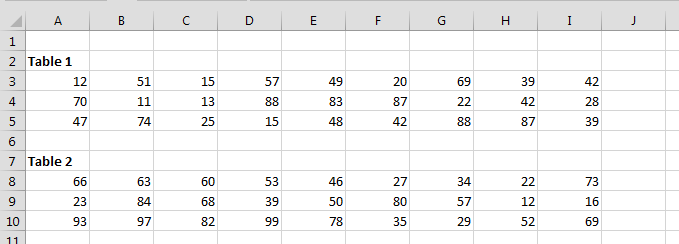To change the value follow below given steps and code:-

• Open the Visual Basic Application
• Enter the below code:-

```Sub Values_1()

With ActiveSheet.UsedRange

Range("A3:I5").Value = Range("A8:I10").Value

End With

End Sub
```

• Run the code by pressing F5
• Values will get updated